## HEY STUDENTS: TEST YOUR KNOWLEDGE OF EQUIVALENT FRACTIONS. TAKE A QUICKIE QUIZ!

Dear Students: Here's your chance to test your knowledge of equivalent fractions. There are five questions. On Questions 1 - 3 choose the picture or figure that shows the fraction named or its equivalent. On Questions 4 and 5 choose the fraction that answers the question.

Some of the questions are easy; others are more difficult. Don't get discouraged. As long as you learn more about equivalent fractions, you'll be a winner!

Question 1: Which circle shows 5/9 of its area shaded blue?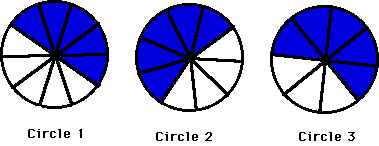The circle that has 5/9 of its area shaded blue is:

Circle 1

Circle 2

Circle 3

Question 2: Which figure shows 3/4 of its area shaded pink?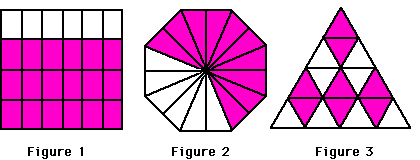The figure that has 3/4 of its area shaded pink is:

Figure 1

Figure 2

Figure 3

Question 3: Which figure shows 1/2 of its circles shaded green?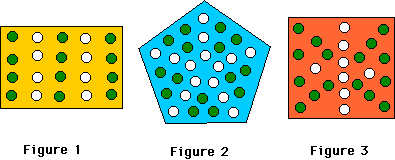The figure that has 1/2 of its circles shaded green is:

Figure 1

Figure 2

Figure 3

Question 4: What fraction of the light bulbs are lit?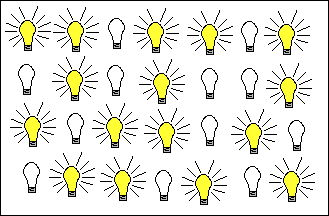a.5/8

b.4/7

c.5/9

Question 5: What fraction of the design is shaded purple?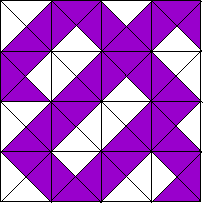b.9/16

c.5/8

SCORING

Score one point for each question that you got correct.

Score a Bonus Point if you took the time to read the answers and explanations.